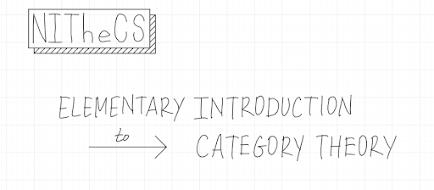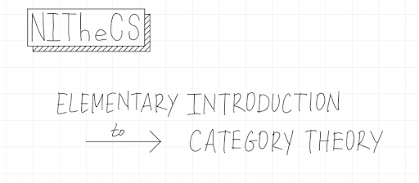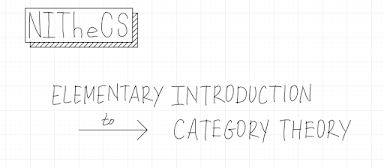## Posts

### Lecture 4: Natural TransformationsThis is the last lecture from NITheCS October 2021 Mini-School. 4.1. The Notion of a Natural Transformation Consider functors F and G between two categories, denoted by blackboard bold C and blackboard bold D: A natural transformation from the functor F to the functor G is defined as follows. Let us unpack this definition. As every other mathematical idea, a natural transformation consists of a "structure" having a certain "property". The structure of a natural transformation is given by a system of arrows in the target category (the target category of functors F and G). This system of arrows is parametrized by objects of the source category. For each object "t" of the source category, there is an associated arrow in the category (blackboard bold) D. As shown above, the source of that arrow is the object F(t) in D, and the target is G(t). This system of arrows must have the following property in order for it to be called a natural transformation from t

### Lecture 3: FunctorsSee exercises below for clarification of some of the discussions during the video lecture, especially the discussions at the end of the lecture. 3.1. An Abstract Notion of a Dynamical System We begin this lecture with an abstract notion of a dynamical system. After recalling this notion, we will try to express it using the language of categories. This process will lead us to a general notion of a mapping between categories: the notion of a functor. A dynamical system is a mathematical structure that consists of a set "X" of states (of a real-life system being modeled), and for each time laps represented by a (non-negative) real number "t", a mapping of members of "X" to members of "X", i.e., a function from the set "X" to the set "X", which we will write as "f" with a subscript "t". The effect of this function on members of the set "X", i.e., on the states, represents the following. For each st

### Lecture 2: ProductsCheck out the exercises for Lecture 2 (not included in the video lecture) at the end of this post. The rest of the post is mostly based on the video lecture. 2.1. The Big Secret We ended last lecture saying that we should discuss examples of categories, as the next step. The two examples we had in mind had to do with the divisibility relation between numbers and composition of functions. Before discussing these examples, let us step back a bit and ask the question: what is the broad intuition behind the concept of a category -- what sort of phenomena of life/nature/science is it supposed to represent? Let us first answer this question for the concept of a directed graph. Directed graphs arise in life/nature/science as situations where a certain type of objects is identified and we would like to investigate a certain type of relation between these objects. For instance, the objects in question could be natural numbers (i.e., measures of whole quantities) and the relation we may be int